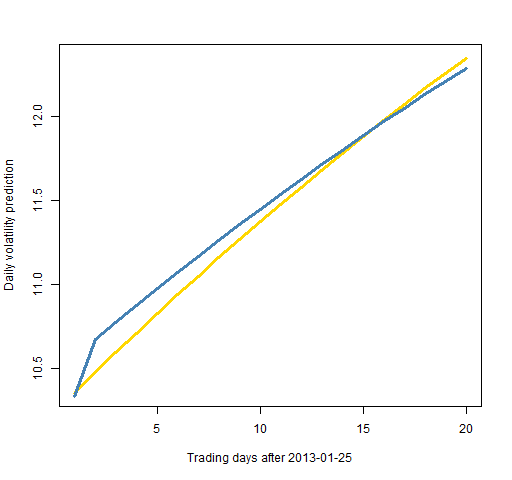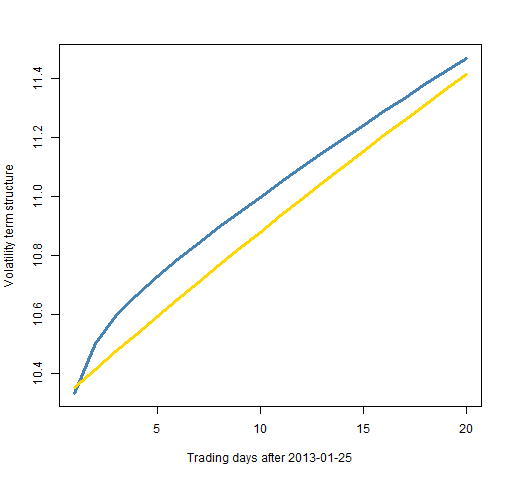Want to share your content on R-bloggers? click here if you have a blog, or here if you don't.

How to fit and use the components model.

## Previously

Related posts are:

## The model

The components model (created by Engle and Lee) generally works better than the more common garch(1,1) model.  Some hints about why it is better are in “3 realms of garch modelling”.

Figure 1 shows predictions of volatility for each day 20 days into the future for two models fit on the S&P 500.  The components model has more nuanced predictions of volatility.

Figure 1: Volatility predictions for each day with the components model (blue) and the garch(1,1) with variance targeting (gold).It is quite possible that this is not the question you would really like answered.  Often what you care about is the average volatility from now until the appointed day.  This is sometimes referred to as the term structure — Figure 2 shows this.

Figure 2: Predicted volatility term structure for the S&P 500 using the components model (blue) and the garch(1,1) with variance targeting (gold).The code to create these plots is shown below.

## R code

The `rugarch` package allows the option of fitting the components model.

### An error

We’ll get to how to do things presently, but first here is a problem I ran into:

```> spx.garct <- ugarchfit(comtspec, tail(spxret, 2000))
Error in .hessian2sidedcpp(f, ipars[estidx, 1], arglist = arglist) :
SET_VECTOR_ELT() can only be applied to a 'list', not a 'symbol'
> packageVersion('rugarch')
 ‘1.0.16’```

I knew what to do after seeing this error because I read the r-sig-finance mailing list.  The hypothesis was that changes to `Rcpp` and relatives probably caused this problem.  So updating those packages should do the trick.

```> detach(package:Rcpp, unload=TRUE)
Error: package ‘Rcpp’ is required by ‘rugarch’ so will not be detached

After unloading the packages, I updated the `Rcpp` and `RcppArmadillo` packages.  Things still didn’t work right, so I did the same exercise of updating `numDeriv` as well.  Then I had different weird behavior.

It turned out there was still a problem with the installation of `Rcpp`.  After installing `Rcpp` once more, there was success.

If I hadn’t seen the r-sig-finance message with a similar error, then my strategy probably would have included doing a web search on a portion of the error message.  In this case the r-sig-finance thread would have shown up.

### Onwards

The components model is called `"csGARCH"` by `rugarch` and the two extra parameters are called `eta11` (ρ in my notation) and `eta21` (φ).  A specification of it with a t distribution and just a constant mean is:

```comtspec <- ugarchspec(mean.model=list(
armaOrder=c(0,0)), distribution="std",
variance.model=list(model="csGARCH"))```

This specification is used like:

```> spx.garct <- ugarchfit(comtspec, tail(spxret, 2000))
Warning message:
In .makefitmodel(garchmodel = "csGARCH", f = .csgarchLLH, T = T,  :
NaNs produced```

The warning message seems to be about:

```> [email protected]\$condH
 NaN```

I’m not sure what this is, but I suspect it isn’t a tragedy that we don’t have it.

The estimated coefficients and half-life are:

```> coef(spx.garct)
mu        omega       alpha1        beta1
7.524949e-04 1.245354e-06 2.892064e-08 8.937168e-02
eta11        eta21        shape
9.918261e-01 8.934750e-02 6.010112e+00
> halflife(spx.garct)
Transitory  Permanent
0.2870233 84.4534694```

Variance targeting seems not to be implemented (yet).

The specification for the garch(1,1) model with variance targeting is:

```tvtspec <- ugarchspec(mean.model=list(
armaOrder=c(0,0)), distribution="std",
variance.model=list(model="sGARCH",
variance.targeting=TRUE))```

### Prediction

Once the models are fit, you can predict.

```spx.ctvolfore <- ugarchforecast(spx.garct,
100 * sqrt(252)

spx.tvtvolfore <- ugarchforecast(spx.gartvt,
100 * sqrt(252)```

The structure of the forecast output is not exactly intuitive, but the `str` function is your friend.

Once we have the predicted volatility in hand, we can plot it.  The function to produce Figure 1 is:

```  function (filename = "dailyvolpred.png")
{
# placed in public domain 2012 by Burns Statistics

if(length(filename)) {
png(file=filename, width=512)
par(mar=c(5,4, 3, 2) + .1)
}
plot(1:20, spx.tvtvolfore, type='l', lwd=3,
col="gold",
ylab="Daily volatility prediction")
lines(1:20, spx.ctvolfore, type='l',
col='steelblue', lwd=3)
if(length(filename)) {
dev.off()
}
}```

The function for Figure 2 is:

```  function (filename = "volpredterm.png")
{
# placed in public domain 2012 by Burns Statistics

if(length(filename)) {
png(file=filename, width=512)
par(mar=c(5,4, 3, 2) + .1)
}
plot(1:20, sqrt(cumsum(spx.ctvolfore^2)/(1:20)),
type='l', lwd=3, col="steelblue",
ylab="Volatility term structure")
lines(1:20, sqrt(cumsum(spx.tvtvolfore^2)/(1:20)),
type='l', col='gold', lwd=3)
if(length(filename)) {
dev.off()
}
}```

The value for the `mar` parameter was intended to be:

`c(5, 4, 0, 2) + .1`

but somehow escaped notice until I was too lazy to change it.